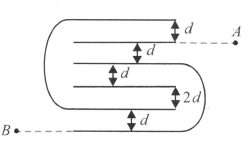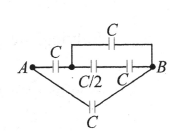Capacitors and capacitance
Question

# Find the equivalent capacitance between A and B. [Assuming each conducting plate is having same dimensions and neglect the thickness of the plate, ${\epsilon }_{0}A/d=7 \mu F,$where A is area of plates and A>>d]Moderate
Solution

## ${C}_{eq}=\frac{\frac{4C}{3}×C}{\frac{7C}{3}}+C=\frac{11C}{7}$Get Instant Solutions